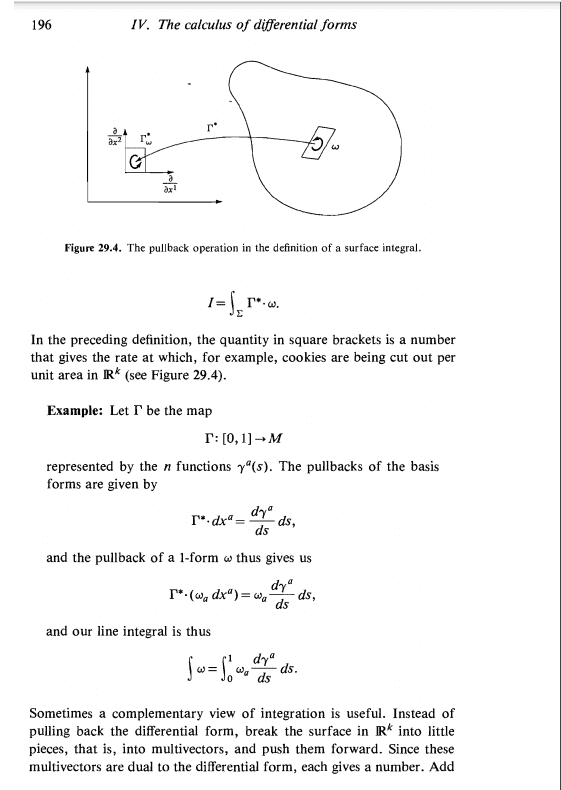# Integration of differential forms

• I
• JonnyG

#### JonnyG

I am confused as to how exactly we integrate differential forms. I know how to integrate them in the sense that I can perform the computations and I can prove statements, but I don't understand how it makes sense. Let's integrate a 1-form over a curve for example:

Let ##M## be a smooth n-manifold, let ##\gamma: [a,b] \rightarrow M## be a smooth curve in M, let ##\omega## be a smooth 1-form on M. Then by definition, $$\int_{\gamma} \omega = \int_{[a,b]} \gamma^{*} \omega$$

Choosing smooth coordinates ##(x^i)## on ##M##, we can write ##\omega = w_i dx^i##. So then by definition, $$\int_{[a,b]} \gamma^{*} \omega = \int_a^b (w_i \circ \gamma) d\gamma^i = \int_a^b (w_i \circ \gamma)\frac{d\gamma^i}{dt} dt$$

From an elementary calculus point of view, this makes sense to me. We take the value of ## (w_i \circ \gamma)\frac{d\gamma^i}{dt} ## at a point on some rectangle of some partition, multiply it by the length of the subrectangle (which is approximated by ##dt##), then we let the mesh of the partition tend to ##0##. The summation makes sense to me because it's a summation of real numbers (the product I just mentioned is a product of real numbers).

Now when I think about this from a differential forms perspective, well ## (w_i \circ \gamma)\frac{d\gamma^i}{dt} ## is a function that is being multiplied by the 1-form ##dt##. The value of ## (w_i \circ \gamma)\frac{d\gamma^i}{dt} ## at a point of a subrectangle is a real number, but the value of ##dt## at a point is a covector, not a real number. So what we really have is a summation of a product of a real number with a covector, which is itself a covector, so how can the result of the integral be a real number?

If I understand you correctly, you are asking about $$\displaystyle{\int_a^b \underbrace{\left(\omega\circ \gamma\right)\cdot \dfrac{d \gamma}{dt} }_{=:F(t)}\,dt}$$
and how length times height can be found in there, if we use the language of differential forms.
The value of ##F(t)## at a point of a subrectangle is a real number, but the value of ##dt## at a point is a covector, not a real number.
You evaluated ##F(t)## at ##t## in that description, but refused to do the same for the second factor ##dt##. A covector is a function which assigns a real number to a vector, i.e. a direction. So if you evaluate both, you get a product of real numbers summed up along the line from ##t=a## to ##t=b##.

I'm not sure whether I like this picture or call it far fetched. One could as well interpret the ##dt## as pure symbolic sign meant to describe what runs in ##\int_a^b##.

Under the keyword exact you can find a specific example here, where I think the multiplications are more transparent. It is function times function and finally summed up over the points (of evaluation).

You evaluated ##F(t)## at ##t## in that description, but refused to do the same for the second factor ##dt##. A covector is a function which assigns a real number to a vector, i.e. a direction. So if you evaluate both, you get a product of real numbers summed up along the line from ##t=a## to ##t=b##.

##dt## is a covector field and evaluated at a point, gives you a covector, which then acts on a tangent vector. So if we are evaluating ##F \cdot dt## at a point ##t_0##, then we get ##F(t_0) dt_{t_0}##, which is a real number multiplied by a covector. I don't see how it makes sense to sum a bunch of covectors to obtain a real number. I hope that clears up my question.

I'm not sure whether I like this picture or call it far fetched. One could as well interpret the ##dt## as pure symbolic sign meant to describe what runs in ##\int_a^b##

But then this is just the usual thing you're told in elementary calculus: the ##dt## tells you what variable to integrate over, so then it seems kind of pointless to have created the theory of differential forms. Differential forms are supposed to be giving meaning to the ##dt##, but with this viewpoint I am just circling back to "it's the variable to integrate over".

##dt## is constantly ##1## along the integration path from ##t=a## to ##t=b##.
But then this is just the usual thing you're told in elementary calculus: the ##dt## tells you what variable to integrate over, so then it seems kind of pointless to have created the theory of differential forms. Differential forms are supposed to be giving meaning to the ##dt##, but with this viewpoint I am just circling back to "it's the variable to integrate over".
Sometimes a cigar is just a cigar. Differential forms give a meaning: The differential form we actually integrate is ##\omega##. That means we have to look for an answer in ##\int_\Gamma \omega ##. As soon as we break it down to a path, we are back at school and ##dt \equiv 1## along this path.

##dt## is constantly ##1## along the integration path from ##t=a## to ##t=b##.

Do you mean that ##dt## is constantly ##1## in coordinates ? If that's what you mean, I don't see how that resolves my misunderstanding: Evaluating ##F \cdot dt## at the point ##t = t_0## gives me the product of a real number with a covector.

But then this is just the usual thing you're told in elementary calculus: the ##dt## tells you what variable to integrate over, so then it seems kind of pointless to have created the theory of differential forms. Differential forms are supposed to be giving meaning to the ##dt##, but with this viewpoint I am just circling back to "it's the variable to integrate over".
People have defined differential forms as "the things you integrate", so don't be alarmed. I've attached an example from a book called "Applied Differential Geometry" by William Burke that might help make sense of why it works.•etotheipi